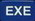5 replies to this topic

### #1Cegafi

Cegafi

Newbie

•• Members
•• 2 posts

Posted 27 October 2010 - 05:01 AM

Hola. Â¿CÃ³mo puedo simplificar (x^5)^(1/2) para que la calculadora me de como resultado (x^2)(x^(1/2))? Gracias por su ayuda.

How can I simplify (x^5)^(1/2) . I want to get (x^2)(x^(1/2)). Or how can I simplify (81*x^7*y^11)^(1/3). Thanks for your help.

Edited by Cegafi, 27 October 2010 - 05:10 AM.

### #2Guest_Casio warrior_*

Guest_Casio warrior_*
•• Guests

Posted 27 October 2010 - 08:54 PM

(x^5)^(1/2)=x^2.5=(x^2)(x^(1/2)) by the laws of exponents which are found in any algebra book.
Similiarly (81*x^7*y^11)^(1/3)=81^(1/3)*x^(7/3)*y^(11/3)

### #3Cegafi

Cegafi

Newbie

•• Members
•• 2 posts

Posted 28 October 2010 - 05:52 PM

Thanks, but I want to know how to get the correct answer in the classpad. I know the laws of exponents. But I can't get it in the classpad.

### #4MicroPro

MicroPro

Casio Overlord

•• Deputy
•• 640 posts
• Gender:Male
• Location:Iran

• Calculators:

Posted 29 October 2010 - 02:51 PM

Hi.
You didn't mention how much you know about classpad so i try to be as clear as possible.
When you enter a variable such as x the classpad doesn't know whether it is positive or negative. This is important when you want to work with radical signs and roots in a calculator. So replace x with abs(x) (select x, go to 2d tab of keyboard and tap || icon). Any of the functions collect, combine, factor, simplify, etc can simplify that expression for you.

For example go to Main and type this: simplify((abs(x)^5)^(1/2))EXE' />
collect(81*(abs(x))^7*(abs(y))^11)^(1/3)EXE' />
simplify((81*(abs(x))^7*(abs(y))^11)^(1/3)EXE' />

Edited by MicroPro, 29 October 2010 - 03:03 PM.

### #5william turner

william turner

Newbie

•• _Members
•• 1 posts

Posted 04 November 2011 - 12:19 PM

the simplify radicals are belong to quadratic equation that simplify the major and minor root cubic root and eliminate the radicals the given example help to solve the simplify the radicals
Simplest radical form means simplifying a radical so that there are no more square roots, cube roots, 4th roots and such left to find. It also means removing any radicals in the denominator of a fraction.

For example, look at √8

Since 8 = 4 * 2

√8 = √(4 * 2)

Since 4 = 2 * 2 it is a perfect square. So take the square root of that number, and thereby take it out of the radical. In other words,
√2 * √2 = 2

So then:

√8 = √(4 * 2) = √2 * √2 * √2

= 2√2

When you solve a problem using the quadratic formula, you are being asked to use this type of method of simplifying.for kind of problem related to algebra and radical expression visit the link.

#### 0 user(s) are reading this topic

0 members, 0 guests, 0 anonymous users